#Function Repository Resource:

# SimplexBoundary

Find the topological boundary of a simplex or simplicial complex

Contributed by: Richard Hennigan (Wolfram Research)
 ResourceFunction["SimplexBoundary"][simplex] finds the topological boundary of simplex. ResourceFunction["SimplexBoundary"][{simplex1,simplex2,…}] finds the topological boundary of the simplicial complex containing simplex1,simplex2,…. ResourceFunction["SimplexBoundary"][mesh] finds the topological boundary of the MeshRegion mesh. ResourceFunction["SimplexBoundary"][complex,k] finds the topological boundary of the k-skeleton of complex.

## Details and Options

A simplex can be considered any of the following:
 Point[v] point Line[{v1,v2}] line segment Triangle[{v1,v2,v3}] or Polygon[{v1,v2,v3}] filled triangle Tetrahedron[{v1,v2,v3,v4}] filled tetrahedron Simplex[{v1,v2,…,vn}] an (n-1)-dimensional simplex
A simplicial complex is either a list of simplices or a MeshRegion where all cells are valid simplices.
The boundary preserves orientations so that it can be used for simplicial homology computations.

## Examples

### Basic Examples (4)

Retrieve the ResourceFunction:

 In:=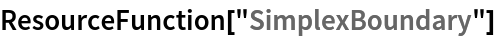Out=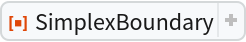Get the boundary of a Simplex:

 In:=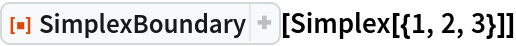Out=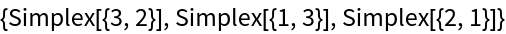Get the boundary of a simplicial complex, represented as a list of simplices:

 In:=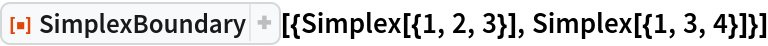Out=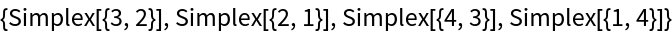Get the boundary of a MeshRegion:

 In:=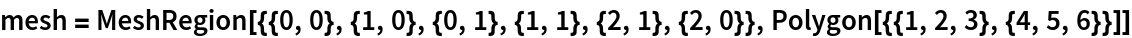Out=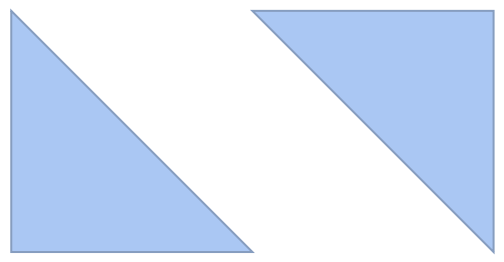In:=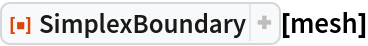Out=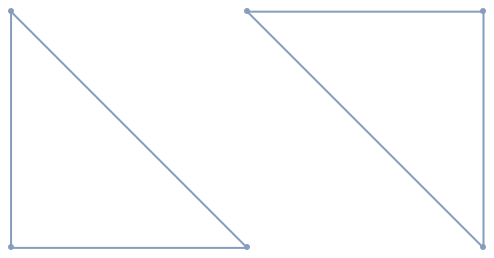### Scope (2)

By default, the boundary will be computed from simplices that match the dimension of the simplicial complex:

 In:=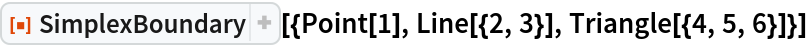Out=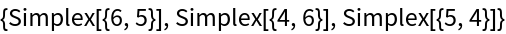A different dimension k can be specified, which will find the boundary of the k-skeleton of the complex:

 In:=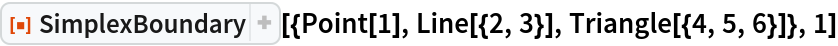Out=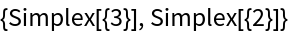### Generalizations and Extensions (1)

Some other primitives can represent a simplex as well:

 In:=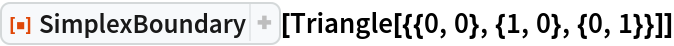Out=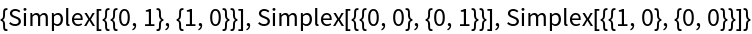In:=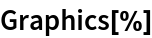Out=### Applications (2)

Find holes in a 3D model:

 In:=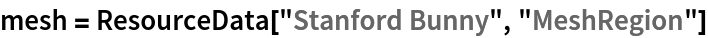Out=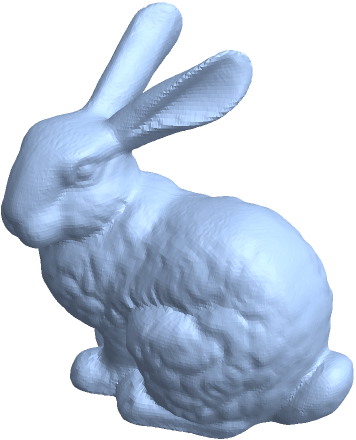In:=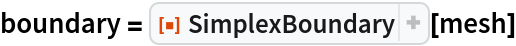Out=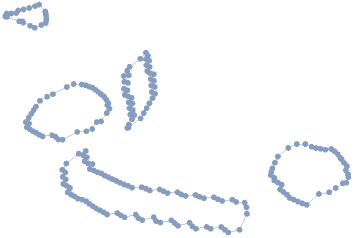Highlight the boundary edges:

 In:=Out=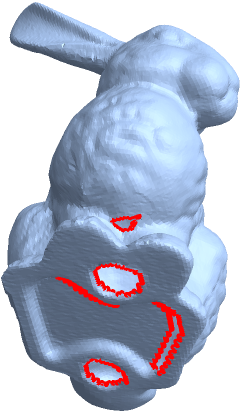### Properties and Relations (3)

Orientation is preserved:

 In:=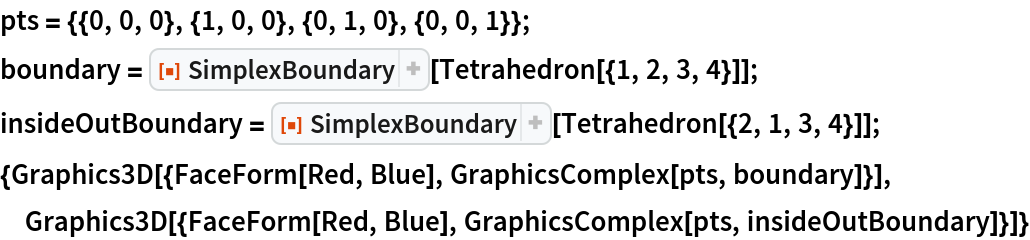Out=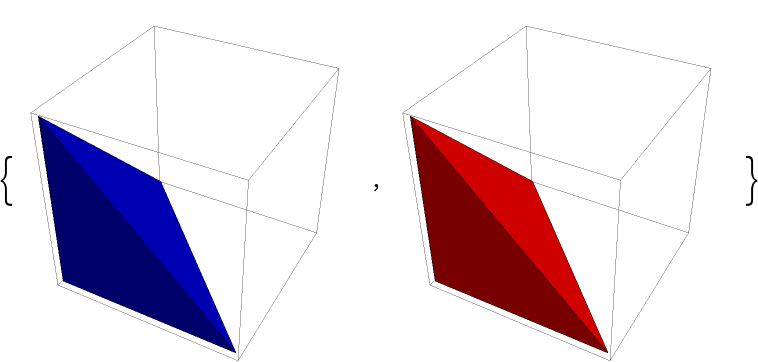In:=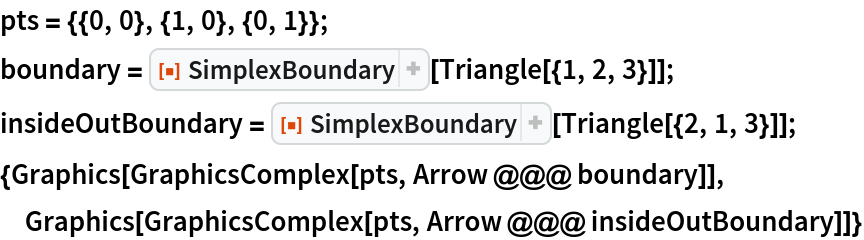Out=The boundary of a boundary is always empty:

 In:=Out=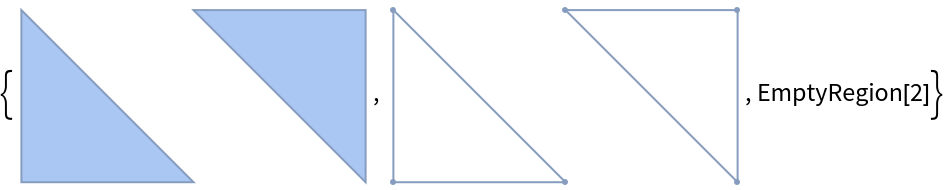In:=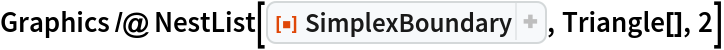Out=In:=Out=A graph can be considered a one-dimensional simplicial complex:

 In:=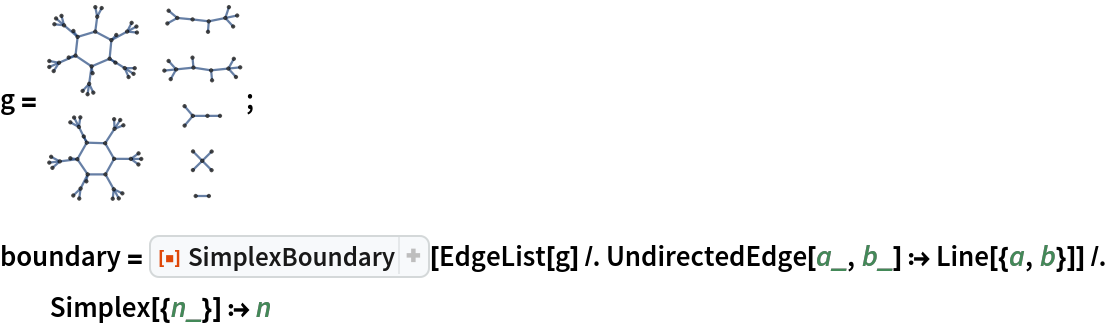Out=In:=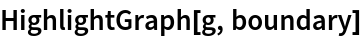Out=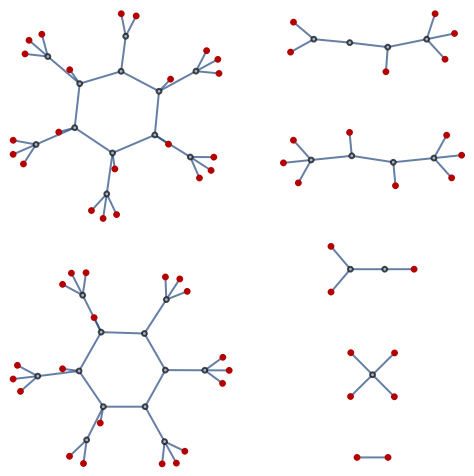The boundary can give you information about degree of vertices:

 In:=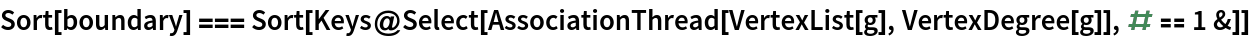Out=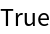### Possible Issues (5)

Not all graphics primitives are valid simplices:

 In:=Out=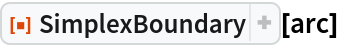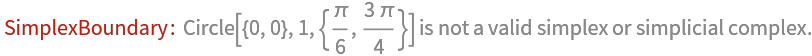Out=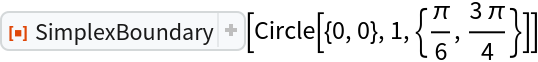They can often be converted to simplicial complexes using DiscretizeGraphics that are reasonable approximations:

 In:=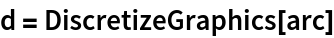Out=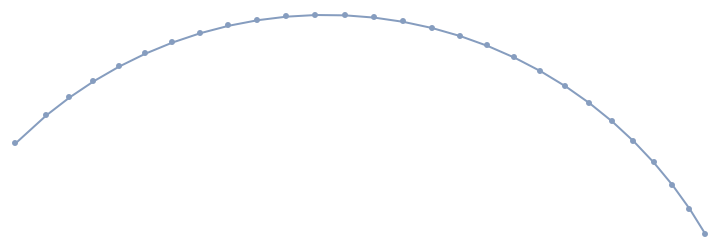In:=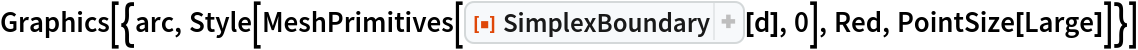Out=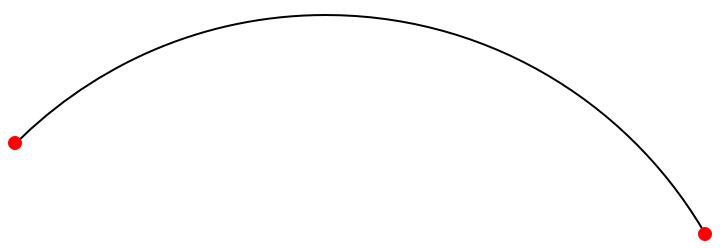Valid n-dimensional simplices must have n+1 vertices: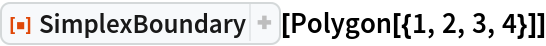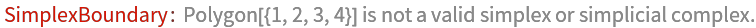Out=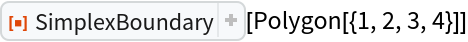In:=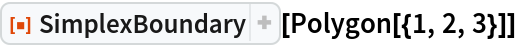Out=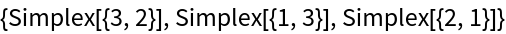Mesh regions are not necessarily composed of simplices:

 In:=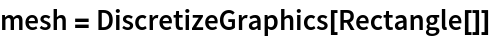Out=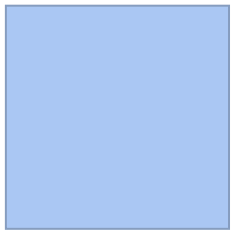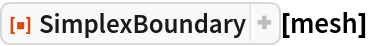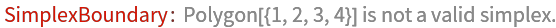Out=TriangulateMesh can often be used to create a valid simplicial complex:

 In:=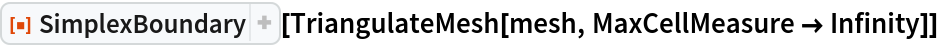Out=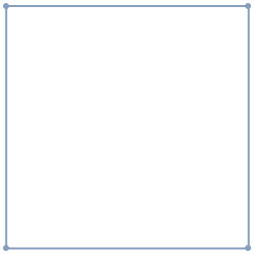Vertices must be unique: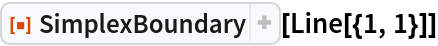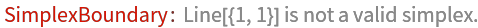Out=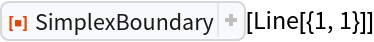If orientations are not consistent within a simplicial complex, the boundary will not have consistent orientations, either:

 In:=Out=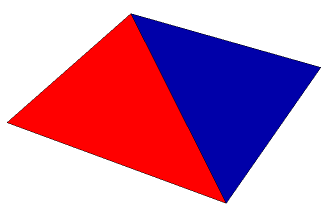In:=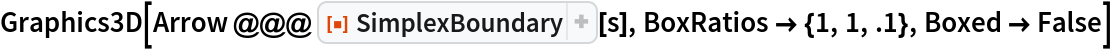Out=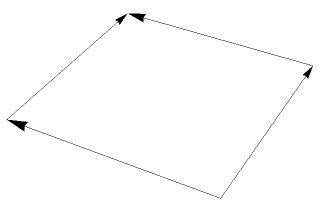### Interactive Examples (1)

One can visualize 4D shapes by rotating and projecting the boundary into 3D. Here is an example using a hexadecachoron:

 In:=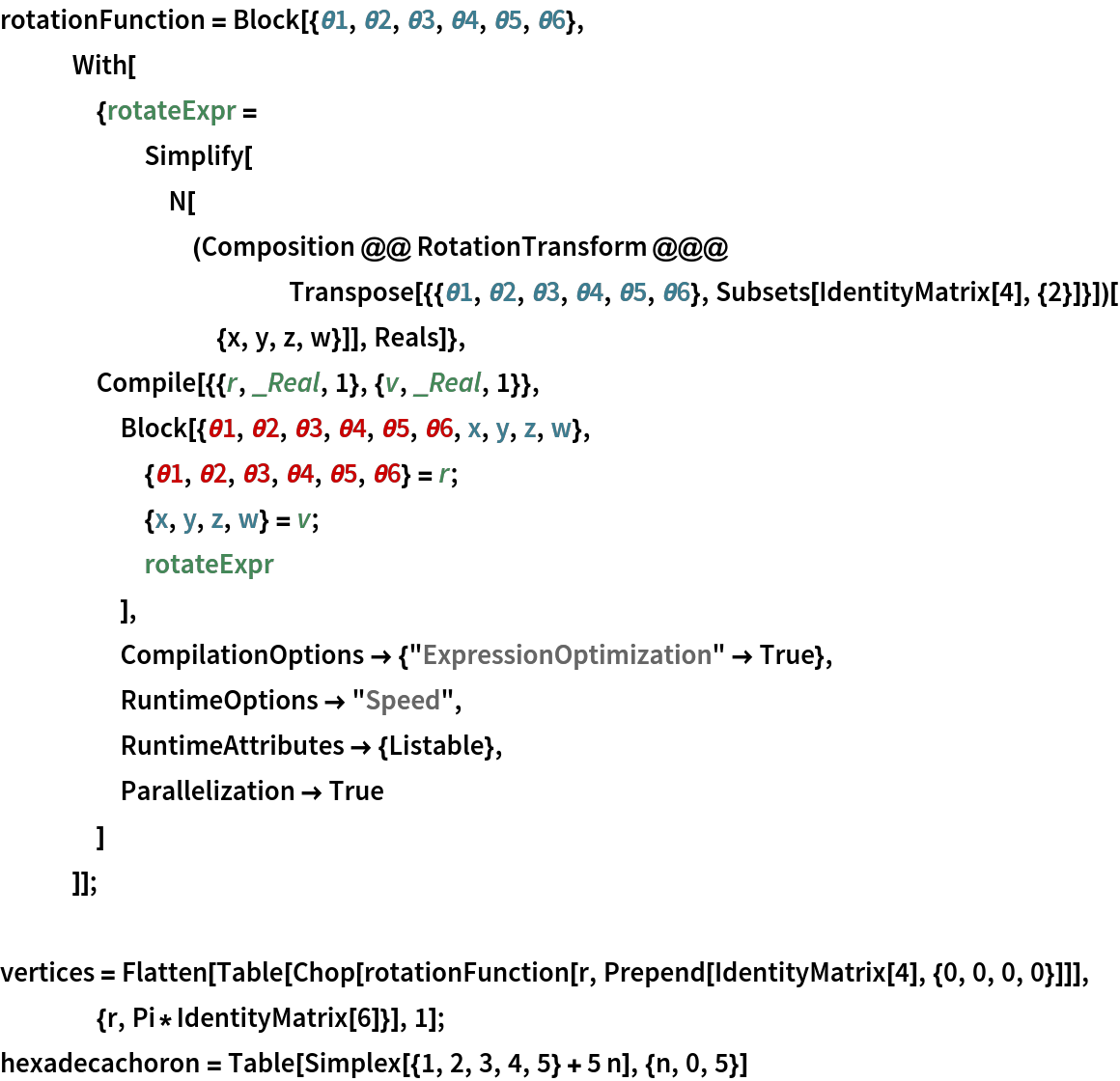Out=In:=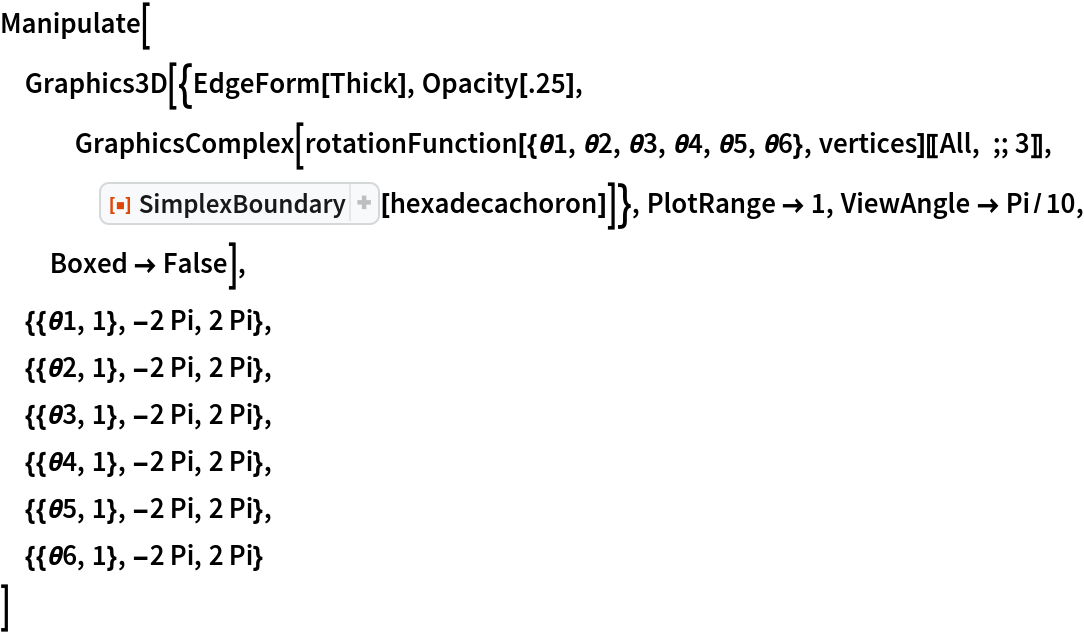Out=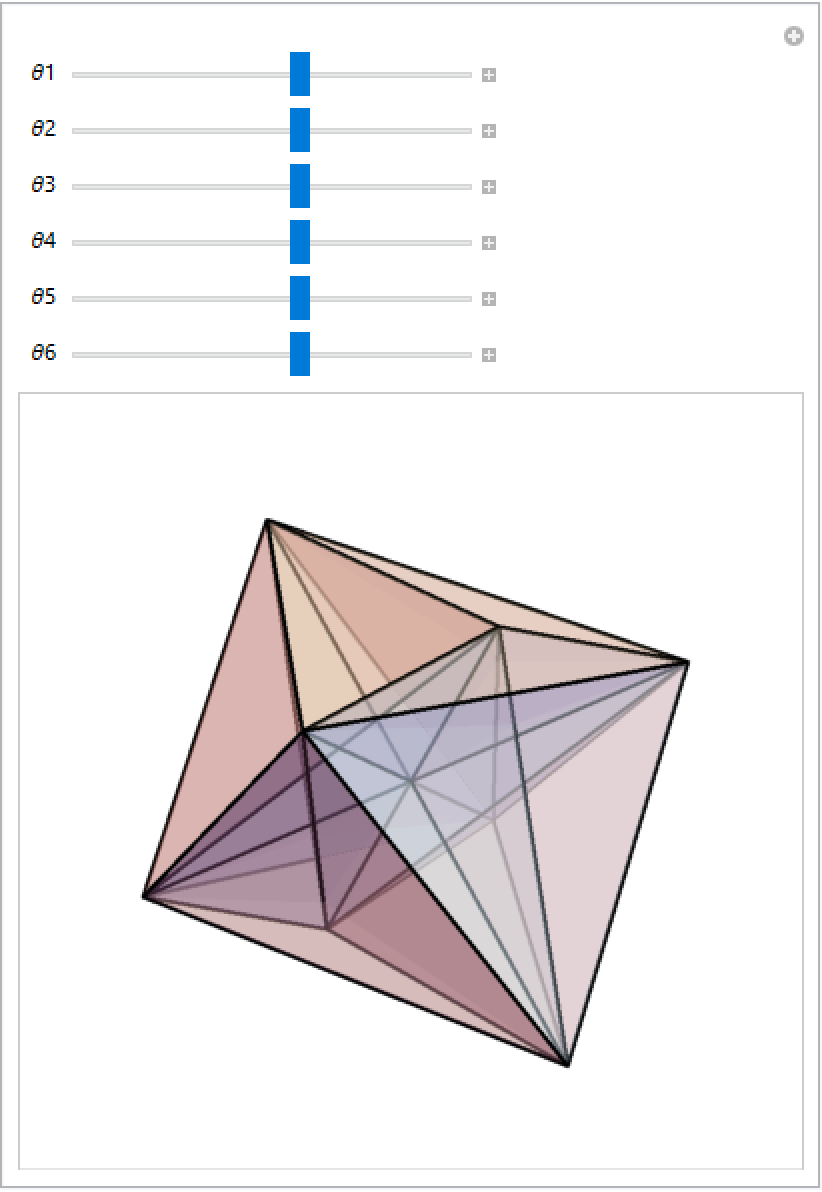### Neat Examples (2)

The boundary of an annulus is two circles, while the boundary of a Moebius strip is a single circle:

 In:=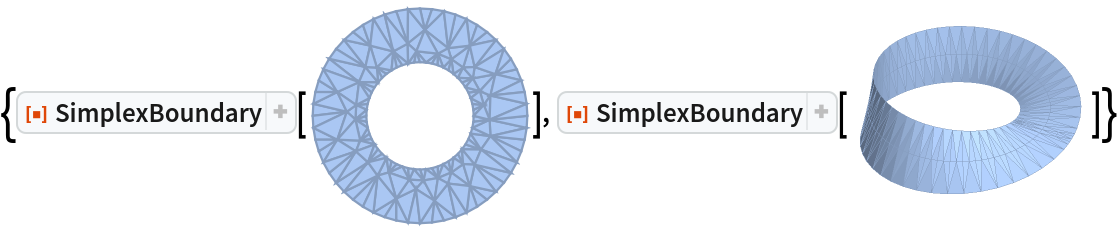Out=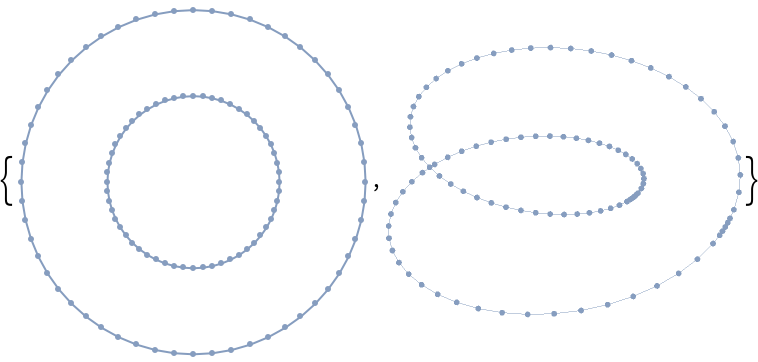Get text outlines:

 In:=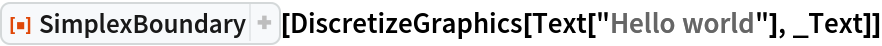Out=## Requirements

Wolfram Language 11.3 (March 2018) or above